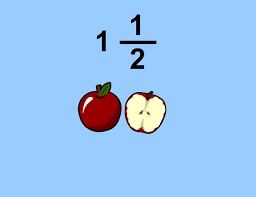# Quotient of two fractions

If the quotient of 8/9 and 1/3 is subtracted from the product of 2 3/4 and 1 3/5, what is the difference? Write the solution as a mixed number or a fraction in the lowest terms.

x =  -26/15

### Step-by-step explanation:Did you find an error or inaccuracy? Feel free to write us. Thank you!

Tips for related online calculators
Need help calculating sum, simplifying, or multiplying fractions? Try our fraction calculator.
Need help with mixed numbers? Try our mixed-number calculator.